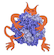IMP  2.3.1 The Integrative Modeling Platform
IMP::algebra::Transformation3D Class Reference

Simple 3D transformation class. More...

#include <IMP/algebra/Transformation3D.h>Inheritance diagram for IMP::algebra::Transformation3D:

## Detailed Description

Simple 3D transformation class.

The rotation is applied first, and then the point is translated.

Definition at line 30 of file Transformation3D.h.

## Public Member Functions

Transformation3D ()
construct an invalid transformation More...

Transformation3D (const Rotation3D &r, const Vector3D &t=Vector3D(0, 0, 0))

Transformation3D (const Vector3D &t)

Transformation3D get_inverse () const

bool get_is_valid () const

const Rotation3Dget_rotation () const
returns the rotation associated with this transformation More...

Vector3D get_transformed (const Vector3D &o) const
transform More...

const Vector3Dget_translation () const
returns the translation vector associated with this transformation More...

Vector3D operator* (const Vector3D &v) const
apply transformation (rotate and then translate) More...

Transformation3D operator* (const Transformation3D &tr) const

const Transformation3Doperator*= (const Transformation3D &o)

Transformation3D operator/ (const Transformation3D &b) const

const Transformation3Doperator/= (const Transformation3D &o)

void show (std::ostream &out=std::cout) const

## Constructor & Destructor Documentation

 IMP::algebra::Transformation3D::Transformation3D ( )

construct an invalid transformation

Definition at line 34 of file Transformation3D.h.

 IMP::algebra::Transformation3D::Transformation3D ( const Rotation3D & r, const Vector3D & t = Vector3D(0, 0, 0) )

basic constructor

Definition at line 36 of file Transformation3D.h.

 IMP::algebra::Transformation3D::Transformation3D ( const Vector3D & t )

Construct a transformation with an identity rotation.

Definition at line 39 of file Transformation3D.h.

## Member Function Documentation

 bool IMP::algebra::Transformation3D::get_is_valid ( ) const
Returns
true if the 3D transformation is valid; false if the transformation was initialized only with the empty constructor, or it was initialized with an invalid rotation.

Definition at line 88 of file Transformation3D.h.

 const Rotation3D& IMP::algebra::Transformation3D::get_rotation ( ) const

returns the rotation associated with this transformation

Definition at line 73 of file Transformation3D.h.

 Vector3D IMP::algebra::Transformation3D::get_transformed ( const Vector3D & o ) const

transform

Definition at line 43 of file Transformation3D.h.

 const Vector3D& IMP::algebra::Transformation3D::get_translation ( ) const

returns the translation vector associated with this transformation

Definition at line 76 of file Transformation3D.h.

 Vector3D IMP::algebra::Transformation3D::operator* ( const Vector3D & v ) const

apply transformation (rotate and then translate)

Definition at line 47 of file Transformation3D.h.Here is the call graph for this function:

 Transformation3D IMP::algebra::Transformation3D::operator* ( const Transformation3D & tr ) const

compose two rigid transformation such that for any vector v (rt1*rt2)*v = rt1*(rt2*v)

Definition at line 50 of file Transformation3D.h.Here is the call graph for this function:

 Transformation3D IMP::algebra::Transformation3D::operator/ ( const Transformation3D & b ) const

Compute the transformation which, when composed with b, gives *this. That is a(x)== d(b(x)) for all x.

For consistency, this should probably have a nice name, but I don't know what name to give it.

Definition at line 63 of file Transformation3D.h.Here is the call graph for this function:

The documentation for this class was generated from the following file: